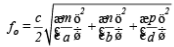# Microwave Measurement Devices Notes | Study Basic Electronics Engineering for SSC JE (Technical) - Electrical Engineering (EE)

## Electrical Engineering (EE): Microwave Measurement Devices Notes | Study Basic Electronics Engineering for SSC JE (Technical) - Electrical Engineering (EE)

The document Microwave Measurement Devices Notes | Study Basic Electronics Engineering for SSC JE (Technical) - Electrical Engineering (EE) is a part of the Electrical Engineering (EE) Course Basic Electronics Engineering for SSC JE (Technical).
All you need of Electrical Engineering (EE) at this link: Electrical Engineering (EE)

Microwave Measurement Devices

Slotted Line: It is used to measure standing wave ratio.
The slot in slotted line is cut at the center, because maximum field will be induced to penetrate probe.

Tunable detector: It helps to detect the low frequency square wave modulated microwave signal.

VSWR meter: It is used to measure VSWR. This meter is not useful for VSWR > 10

Power meter: It is used to measure microwave power.
It has power sensor that converts power into heat energy. Temperature change provides an output current that indicates power.

Wave meter: It is used to measure frequency of microwaves in a system by selective basorption of microwave energy in solids.

Resonant frequencySpectrum Analyzer: It gives a display of frequency spectrum of input signal. (Gives a plot of signal amplitude vs time)

Network Analyzer : It measures both amplitude an phase of microwave signal over a wide frequency range.

Measurement of Power: 

• Low power (0.01 mW – 10 mW) ® Bolometer technique
• Medium power ( 10 mW – 10 W) ® Calorimetric technique 
• High power (> 10 W) ® Calorimetric technique
The document Microwave Measurement Devices Notes | Study Basic Electronics Engineering for SSC JE (Technical) - Electrical Engineering (EE) is a part of the Electrical Engineering (EE) Course Basic Electronics Engineering for SSC JE (Technical).
All you need of Electrical Engineering (EE) at this link: Electrical Engineering (EE)Use Code STAYHOME200 and get INR 200 additional OFF

## Basic Electronics Engineering for SSC JE (Technical)

25 docs|22 tests

Track your progress, build streaks, highlight & save important lessons and more!

,

,

,

,

,

,

,

,

,

,

,

,

,

,

,

,

,

,

,

,

,

;